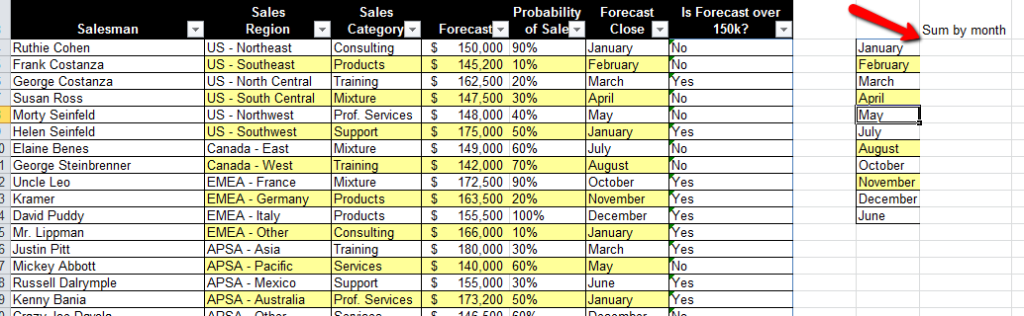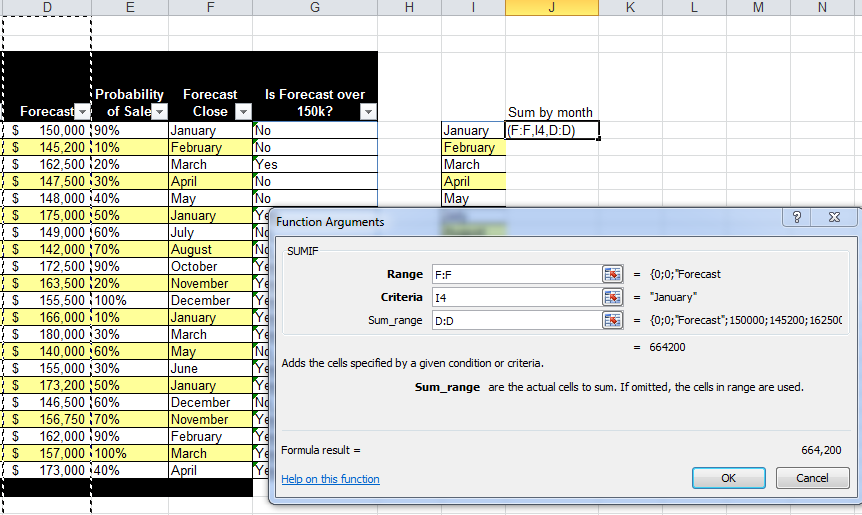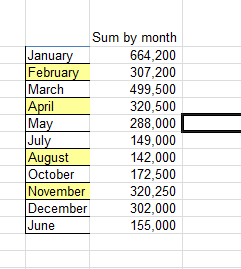# Sumif function

The SUMIF function will look for certain criteria and if it finds it, then it will Sum up related cells.

For example:Here we have a table of salesmen with forecasts and month of their forecast close.  On the right, we listed the months and we want to sum up the Forecasts for each month.  So go to the first cell and insert the SUMIF functionRange – This is a group of cells that we want to look in.  In this example, we want to find the months in this column

Criteria – This is the cell we are looking for.  This example we use cell I4, which is the month in the right-hand table.  In this example, it’s January.

Sum range – This is the column we need to look in to pull in the dollar amount.  In this example its the Forecast column, which is column D.Then after you copy it down, you will get the results like above.  Then this will change as the values in the original table change.